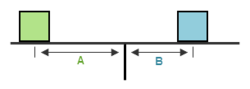## ASVAB Mechanical Comprehension Other Forces Practice Test 240604

Question 1 of 5
###### Torque

Torque measures force applied during rotation: τ = rF. Torque (τ, the Greek letter tau) = the radius of the lever arm (r) multiplied by the force (F) applied. Radius is measured from the center of rotation or fulcrum to the point at which the perpendicular force is being applied. The resulting unit for torque is newton-meter (N-m) or foot-pound (ft-lb).A = 6 ft., the green box weighs 35 lbs., and the blue box weighs 40 lbs. What does distance B need to be for this lever to balance?
 1.75 ft. 240 ft. 2.63 ft. 5.25 ft.